Teaching Physics with the Physics Suite Edward F. Redish Problems Sorted by Type | Problems Sorted by Subject | Problems Sorted by Chapter in UP

Testing the Theorems

 In this problem we will test whether the Impulse-Momentum and Work-Energy theorems actually hold for a real-world example.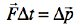(Impulse-Momentum Theorem)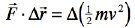(Work-Energy Theorem) We will test it in this problem using our program that allows you to take data from a video. You can download a video here that shows a cart being pulled on a track by a string. The track has very little friction. To do this, we will note that the right hand side on the two theorems are a difference, so we will get them by comparing two values. The ones on the left are products, so we will have to add them up by finding the areas on appropriate graphs. LoggerPro will provide you with the needed tools.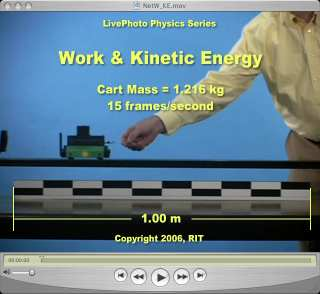a) Draw a free body diagram for the cart going forward. Which forces contribute to the net force on the cart? Which, if any, cancel?

b) Analyze the video in LoggerPro and get an x vs. time, a vx vs. time graph, and an ax vs. time graph. An appropriate LoggerPro file is available for downloading. If you need a reminder on how to use Logger Pro see the instruction document.

c) Since we will need forces, we have to have a Fx vs. time graph. How can you get the net force in the x direction from the data you already have? Find an equation for Fx at a particular instant of time.

Since this Logger Pro file doesn't calculate Fx on its own, you will have to set it up to do so. Begin by adding a new column to calculate F:

• Go up to the top of the window and select "Data" →"New Calculated Column..."
• A window will open where you can name your column "Net Force X " and give it a short name "Fx". Be sure also to type in the proper units so that Logger Pro gives you the correct units.
• At the bottom of the window, you need to set the equation for Fx. You do it by entering "=" and then selecting the relevant variables and parameters for your formula from the drop down boxes at the bottom. To to add, use "+", to subtract use "-", to multiply, use "*", and to divide, use "/" (without the quotes) .
• Click "Done"
• Now you should see the "Fx" column in your data table and you should be able to select "Fx" as one of the choices of variables to graph. Slide your curson on the left of the bottom graph where the axis label should be. Click on the "More..." box that appears and select Fx.

Now we want to use the graphs in Logger Pro to get the appropriate numbers in order to do the calculations. There are two Logger Pro functions which may help. Select the graph you want to analyze and at the top of the screen click "Analyze" or click the toolbar buttons indicated below:

• "Examine"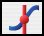- This will give you data on any point on the graph that you put your mouse over
• "Integral"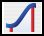- This will give you the area under a part of the data that you have selected by clicking and dragging on a graph.

d) From t = 0.2 s to t = 1.4 s, find

• The impulse, FΔt, exerted on the cart
• The change of momentum, Δp, of the cart
• The work, W, done on the cart
• The change of kinetic energy, ΔKE, of the cart

e) What is the percent difference between FΔt and Δp? between W and ΔKE? Is it reasonable to say that Impulse-Momentum and the Work-Energy theorems actually hold for this cart in this situation?

By Eric Kuo and E. F. Redish

Not finding what you wanted? Check the Site Map for more information.